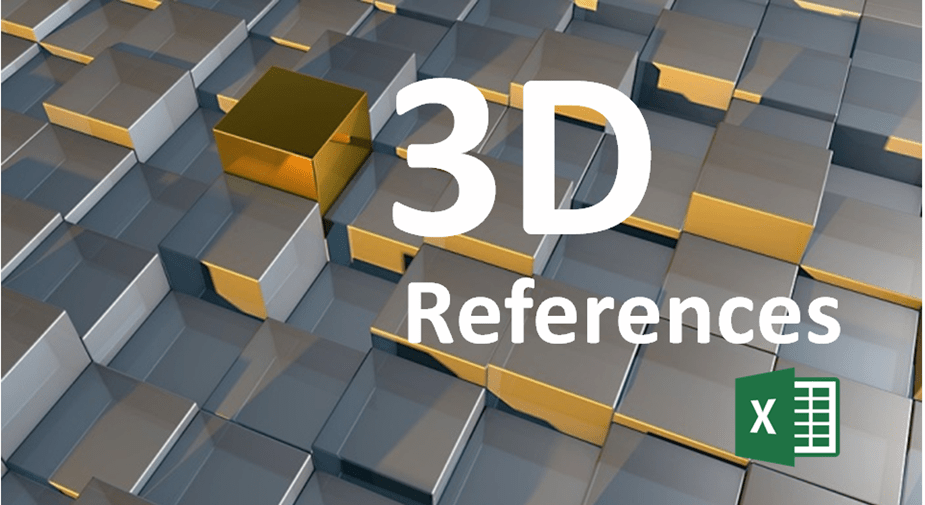## Summarize Multiple Excel Worksheets Using 3D References in Formulas

by Avantix Learning Team | Updated March 2, 2019

Applies to: Microsoft® Excel® 2010, 2013, 2016, 2019 and 365 (Windows)

You can use 3D references in Excel formulas to calculate across multiple worksheets that are structured in the same way. A 3D reference refers to the same cell or range of cells on multiple worksheets.

Recommended article: How to Enter Data in an Excel Filtered List into Visible Cells [2 Ways]

### Working with 3D references

Since a 3D reference refers to the same cell or range of cells on multiple worksheets, it references the cell or range of cells and also a range of worksheet names.

All of the worksheets you wish to reference must be set up the same way and use the same data types.

For example, if you want to create a total for product sales and extract data from January, February, March and April worksheets where the total is in cell B6 on all sheets, you could create the following formula:

=January!B6+February!B6+March!B6+April!B6

However, if you have 12 sheets for the year or even more sheets, you could end up with a fairly long formula.

As an alternative, you could use a 3D reference with the SUM function to total all of the sheets as in the following formula:

=SUM(January:April!B6)

You can also use ranges of cells as in the following 3D formula:

=SUM(January:April!B2:B5)

### Syntax for 3D formulas

The syntax for a 3D formula is:

=Function(Firstsheet:Lastsheet!cell)

or

=Function(Firstsheet:Lastsheet!range)

When you are using 3D formulas in Excel, all worksheets between the first worksheet and the last worksheet are included in the formula.

### Creating formulas with 3D references

To create a formula with a 3D reference:

1. Click the cell where you want to enter a 3D formula (such as a cell on a summary worksheet).
2. Type an equal sign (=), enter the function’s name (such as SUM) and then type an open round bracket.
3. Click the tab of the first worksheet that you want to include in the 3D reference.
4. Press Shift and click the tab of the last worksheet that you want to include in the 3D reference.
5. On the first worksheet, click the cell or drag over the range of cells that you want to include in the formula. The cell or cells must be in the same location on all sheets.
6. Enter the remainder of the formula as required and a closed round bracket.
7. Press Enter to complete the 3D formula. For example, =SUM(January:April!B6).

### How 3D references update when you insert, move or delete worksheets

Because each 3D reference in Excel is defined by the starting and ending worksheet (the 3D reference end points), changing the end points changes the reference which then changes your 3D formula.

If you insert, copy or move worksheets between the starting and ending worksheet, the referenced range in all newly added sheets will be included in the 3D formula.

When you move any of the worksheets outside of the endpoints, these worksheets will be excluded from your 3D formula. If you move the ending worksheet to the start, or the starting worksheet to the end, the sheets will also be excluded.

If you restore the original order of the starting or ending worksheet, this will not restore the original 3D reference. You should recreate the formula.

When you delete the starting or ending worksheet, it is removed from the 3D reference and the deleted endpoint changes. If the first sheet is deleted, the end point changes to the sheet that follows it. If the last sheet is deleted, the end point changes to the preceding sheet.

### Excel functions that allow 3D references

You can use 3D references with the following Excel functions:

SUM

AVERAGE

AVERAGEA

COUNT

COUNTA

MAX

MAXA

MIN

MINA

PRODUCT

STDEV, STDEVA, STDEVP and  STDEVPA

VAR, VARA, VARP and VARPA

Formulas with 3D references are a great way to summarize the same cells or ranges from multiple sheets.

#### Recommended Microsoft Excel training

Microsoft Excel: Data Analysis with Functions, Dashboards and What-If Analysis Tools

Microsoft Excel: Introduction to Visual Basic for Applications (VBA)

VIEW MORE COURSES >

Our instructor-led classroom training courses are delivered at our downtown Toronto location at 1 Yonge Street, Suite 1801 (Toronto Star Building), Toronto, Ontario, Canada (some courses may also be delivered at an alternate downtown Toronto location). Contact us if you’d like to arrange custom training at your office on a date that’s convenient for you.

#### You may also like

Microsoft, the Microsoft logo, Microsoft Office and related Microsoft applications and logos are registered trademarks of Microsoft Corporation in Canada, US and other countries. All other trademarks are the property of the registered owners.

Avantix Learning | 1 Yonge Street, Suite 1801 (Toronto Star Building), Toronto, Ontario, Canada M5E 1W7 | info@avantixlearning.ca

SummaryArticle Name
How to Create 3D References in Microsoft Excel
Description
You can use 3D references in formulas to calculate across multiple worksheets that are structured in the same way. A 3D reference refers to the same cell or range of cells on multiple worksheets.
Author
Publisher Name
Avantix Learning Inc.